# High School Math : Graphing Linear Functions

## Example Questions

### Example Question #1 : Graphing Linear Functions

Solve for the- and- intercepts: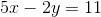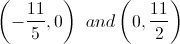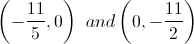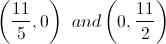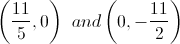Explanation:

To solve for the-intercept, setto zero and solve for: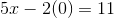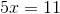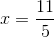To solve for the-intercept, setto zero and solve for: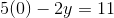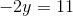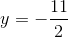### Example Question #2 : Graphing Linear Functions

Solve: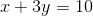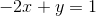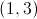Infinitely many solutions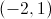No solutions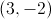becomes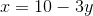and then is substituted into the second equation. Then solve for: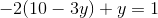, so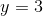andto give the solution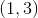.